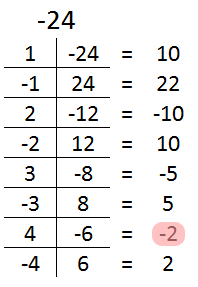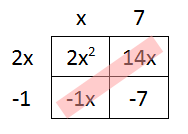Factoring Polynomials
 Home > Lessons > Factoring Polynomials Updated March 26th, 2020
Introduction

In this section, you will learn how to factor various quadratic expressions, which are 2nd degree polynomials. Here are the sections in this lesson:

Before learning how to factor polynomials, you must first understand how to multiply polynomials. If you are not knowledgeable of polynomial multiplication, or addition and subtraction, view this lesson before proceding to the sections that follow.esson: Operations on Polynomials

There important reasons for learning about factors. One important reason is that large prime numbers are used for making the Internet secure. Having secure transactions over the Internet is vital for businesses, governments, and personal data. Here is Dr. Holly Krieger who explains a conjecture using a difference of two squares: Catalan's Conjecture -- Numberphile. [This is not a MATHguide source].

This video will explain how to factor polynomials which contain terms that have common factors.ideo: Factoring: Greatest Common Factoruizmaster: Factoring Binomials for the GCF

This video will explain how to factor binomials that have the form called a difference of two squares.ideo: Factoring: Difference of Two Squares

Try this interactive quizmaster to determine if you understand this lesson.uizmaster: Factoring a Difference of Two Squaresuizmaster: Factoring a Difference of Two Squares with Help

Factoring ax2 + bx + c when a = 1

When attempting to factor quadratics that have a leading coefficient of 1, we must focus on the values of 'b' and 'c.' The systematic approach for factoring involves factoring 'c' in as many different ways as possible into pairs. Then, find the pair that has a sum that amounts to the value of 'b.'

In short, we are looking for a pair of numbers that satisfy two requirements at the same time: they must have a product equal to 'c' and a sum equal to 'b.' Use the examples below to help clarify this technique.

1.Let's start with x2 + 10x + 21. Since c = 21, we will factor 21 in as many pairs as possible. The pairs are: {1, 21} and {3, 7}. Now we must find a pair that has a sum equal to 'b,' which is 10. The pair {1, 21} has a sum of 22, but this does not match our value for 'b.' The pair {3, 7} has a sum of 10, and that is a match!

This means that this trinomial can be factored to (x + 3)(x + 7).

2.Let's take another trinomial that has more possibilities, namely x2 - 2x - 24. This problem is more involved because 'c' has a lot of factors and because there are negatives to juggle. If we make a list of all possible factors of -24, we get: {1, -24}, {2, -12}, {3, -8}, {4, -6}, {6, -4}, {8, -3}, {12, -2}, and finally {24, -1}. Our pairs have sums of -23, -10, -5, -2, 2, 5, 10, and 23, respectively. Our sum {4, -6} is a match, so {4, -6} is the winning combination.

This means x2 - 2x - 24 factors to (x + 4)(x - 6).ideo: Factoring Trinomialsuizmaster: Factoring Simple Quadraticsuizmaster: Factoring Simple Quadratics with Help

Factoring ax2 + bx + c when a > 1

This type of trinomial is much more difficult to factor than the type 1 variety. Instead of factoring the 'c' value alone, one has to also factor the 'a' value. This compounds the number of checks necessary to verify our possible solution. As if this was not enough work, the checking method is much more involved, too.

Our factors of 'a' become coefficients of our x-terms and the factors of 'c' will go right where they did for the type 1 problems, to the right of each binomial.

When we place numbers to form binomials in every possible combination, we then have to check to see which factorization, if any, works. To check, multiple the first coefficient times the right-most right number to get one product and multiply the second coefficient times the left-most right number to get the second product. Add these two products and check to see if this sum is equal to 'b' in the original trinomial.

This checking method is best described and understood graphically, so examine the two examples below for further clarification.

1. Let's take a look at 2x2 + 13x - 7. Our factors of 'a' are: {1, 2} and {2, 1}. Factors of 'c' are: {1, -7} and {7, -1}. Even though there are not many factors for each value, the manner in which we construct our binomials so that every possible combination is made will have us checking many options.

Since the factors of 'a' are coefficients of x and factors of 'c' are the stand-alone numbers, we can get the following possible options:

(x + 1)(2x - 7)
(x + 7)(2x - 1)
(x - 1)(2x + 7)
(x - 7)(2x + 1)

Notice how the coefficients of x were held constant between options and the stand-alone numbers were flipped back and forth so that every possible option could be written.Now there's the check to see if there is a solution here. We multiply the two innermost terms together to form one product and multiply the outermost two terms together to form the second product. Then, we add the products and check the sum with the original 'b-value' of our trinomial to see if we can find a match.

For our pair of binomials, we get 2x and -7x for products and a sum of -5x. The second pair of binomials yields 14x + (-1x) = 13x. Our fourth gives us -2x  + 7x, which is 5x. Our last pair of binomials gives us -14x + 1x = -13x. We can find our match with our second check, so the factors are...

(x + 7)(2x - 1)

2. For our last example, let's look at 6x2 - 19x + 3. There are many combinations here! Factors of a are: {1, 6}, {2, 3}, {3, 2}, and {6, 1}. Factors of 'c' include {1, 3}, {3, 1} {-1, -3}, and {-3, -1}.

Now we have to piece together all possible binomials:

(x + 1)(6x + 3)
(x + 3)(6x + 1)
(x - 1)(6x - 3)
(x - 3)(6x - 1)
(2x + 1)(3x + 3)
(2x + 3)(3x + 1)
(2x - 1)(3x - 3)
(2x - 3)(3x - 1)

Since our original problem has a negative 'b-value,' our possible solutions should be limited to those having negative values within them.So if we only need check the binomial pairs that have negative values, we only need to check these factors.

(x - 1)(6x - 3)
(x - 3)(6x - 1)
(2x - 1)(3x - 3)
(2x - 3)(3x - 1)

This makes only four valid options, total. The checking for the first binomial pair is -6x + (-3x) = -9x. The second has -18x + (-1x) = -19x. The third binomial pair checks to -3x + (-6x) = -9x. The last binomial pair has -9x + (-2x) = -11x. So the pair that works is the second pair (x - 3)(6x - 1).ideo: Factoring Trinomialsuizmaster: Factoring Complex Quadratics

Factoring ax2 + bx + c when a < 1

It is possible to have a polynomial with a < 1, in other words with a leading coefficient less than 1. In the case that our leading coefficient is negative, simply factor out the -1 and use the techniques described above on the resulting trinomial. For example, this trinomial can be factored.

-3x2 + 4x - 1

It first has to be prepared by factoring out a negative 1, like so.

-(3x2 - 4x + 1)

Now, the strategy for factoring trinomials could be used on the trinomial that has a positive leading coefficient.

 Instructional Videos After reading the lessons, try our videos:ideo: Factoring: Greatest Common Factorideo: Factoring: Difference of Two Squaresideo: Factoring: Trinomials Interactive Quizzes After reading the lessons, try our quizmasters. MATHguide has developed numerous testing and checking programs to solidify these skills:uizmaster: Factoring Binomials for the GCFuizmaster: Factoring a Difference of Two Squaresuizmaster: Factoring a Difference of Two Squares with Helpuizmaster: Factoring Simple Quadraticsuizmaster: Factoring Simple Quadratics with Helpuizmaster: Factoring Complex Quadratics Related Lessons MATHguide also has interactive lessons that can demonstrate the need to know how to simplify algebraic exressions:esson: Operations on Polynomialsesson: Solving Complicated Proportionsesson: Algebra Magic 1: Simpleesson: Algebra Magic 2: Intermediateesson: Algebra Magic 3: Intermediateesson: Algebra Magic 4: Advanced